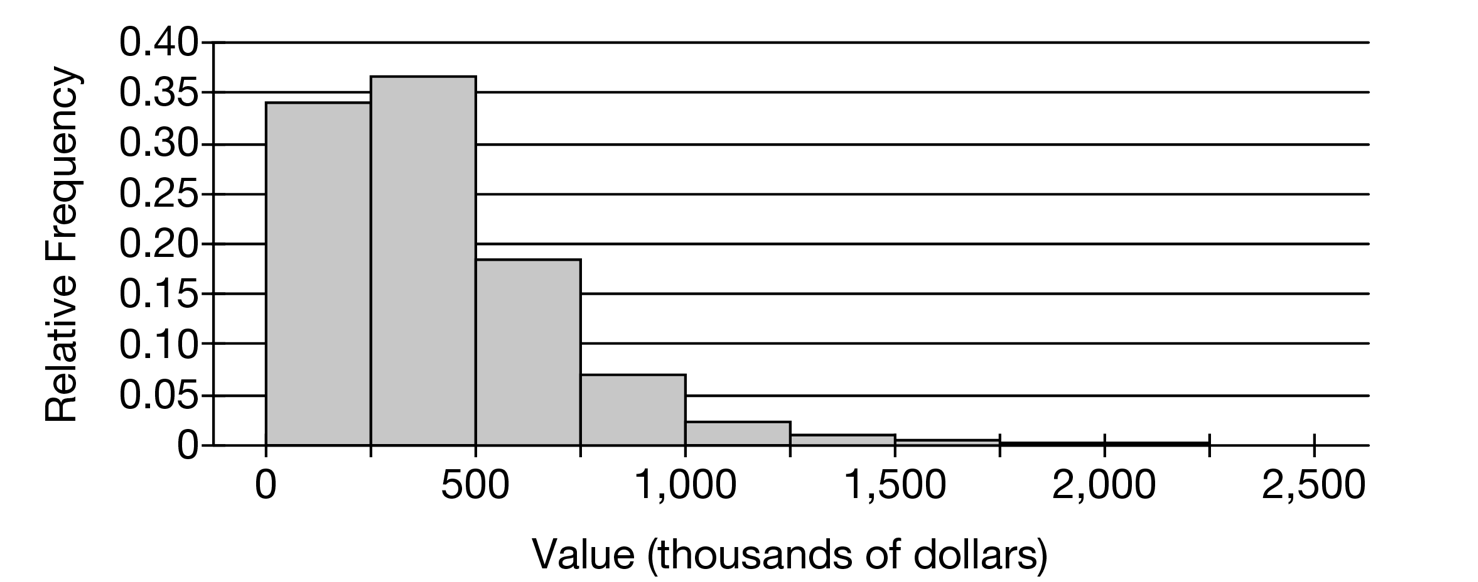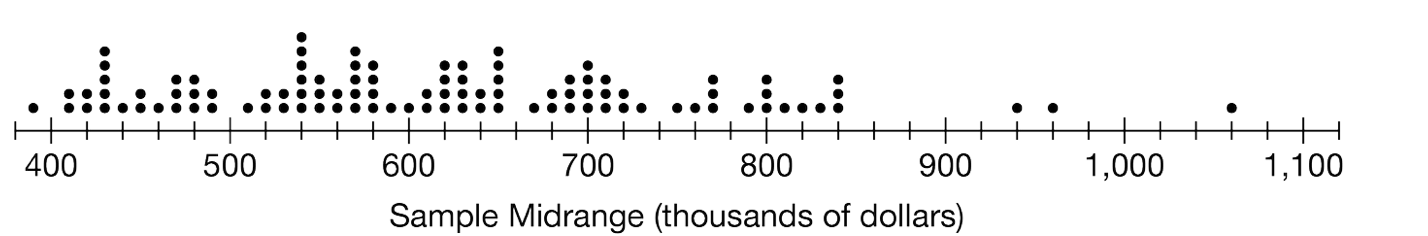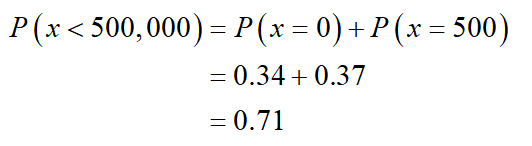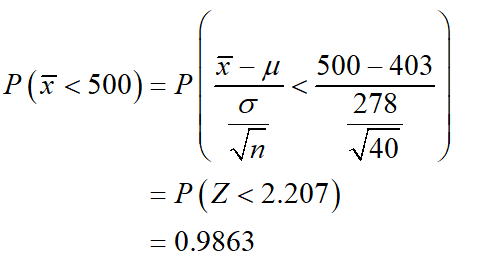# 0.40-0.35-0.30-0.25-0.20-0.150.10-0.05-5001,0001,5002,0002,500Value (thousands of dollars)Relative Frequency :...!4005006007008009001,0001,100Sample Midrange (thousands of dollars)

Question
274 views

The following histogram shows the distribution of house values in a certain city. The mean of the distribution is \$403,000 and the standard deviation is \$278,000.

(a) Suppose one house from the city will be selected at random. Use the histogram to estimate the probability that the selected house is valued at less than \$500,000. Show your work.

(b) Suppose a random sample of 40 houses are selected from the city. Estimate the probability that the mean value of the 40 houses is less than \$500,000. Show your work.

To estimate a population mean μ, the sample mean x¯ is often used as an estimator. However, a different estimator is called the sample midrange, given by the formula sample minimum + sample maximum2.

(c) The following table shows the values, in thousands of dollars, of 40 randomly selected houses in the city.

 34 38 95 110 137 140 155 155 169 207 209 217 274 314 314 323 343 347 349 363 369 373 388 389 397 416 448 450 483 487 488 516 571 595 600 738 762 769 863 1,084

(i) Calculate the sample midrange for the data.

(ii) Explain why the sample midrange might be preferred to the sample mean as an estimator of the population mean.

(d) To investigate the sampling distribution of the sample midrange, a simulation is performed in which 100 random samples of size n=40 were selected from the population of house values. For each sample, the sample midrange was calculated and recorded on the following dotplot. The mean of the distribution of sample midranges is \$617,000 with standard deviation \$136,000.

Based on the results of the simulation, explain why the sample mean might be preferred to the sample midrange as an estimator of the population mean.help_outlineImage Transcriptionclose0.40- 0.35- 0.30- 0.25- 0.20- 0.15 0.10- 0.05- 500 1,000 1,500 2,000 2,500 Value (thousands of dollars) Relative Frequency fullscreenhelp_outlineImage Transcriptionclose:...! 400 500 600 700 800 900 1,000 1,100 Sample Midrange (thousands of dollars) fullscreen
check_circle

Step 1

"Since you have posted a question with multiple sub-parts, we will solve first three sub-parts for you. To get remaining sub-part solved please repost the complete question and mention the sub-parts to be solved."

(a)

The probability that the selected house is valued at less than \$500,000 obtained using the given histogram is,Thus, the probability that the selected house is valued at less than \$500,000 is 0.71.

Step 2

(b)

The probability that the mean value of 40 houses is less than \$500,000 is,Thus, the probability that the mean value of 40 houses is less than \$500,000 is 0.9863.

...

### Want to see the full answer?

See Solution

#### Want to see this answer and more?

Solutions are written by subject experts who are available 24/7. Questions are typically answered within 1 hour.*

See Solution
*Response times may vary by subject and question.
Tagged in